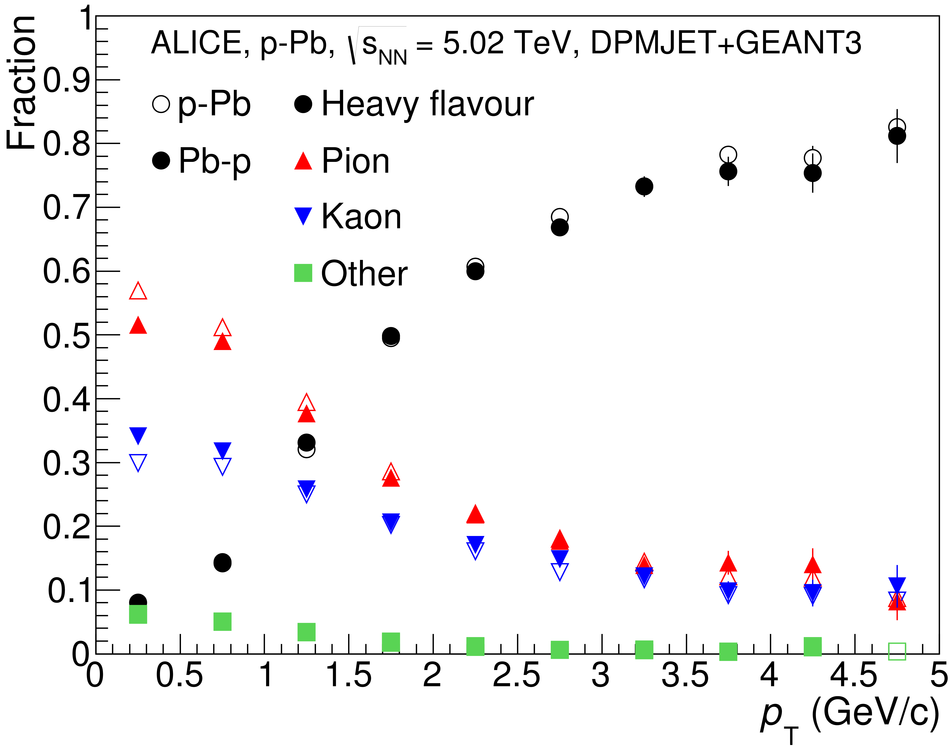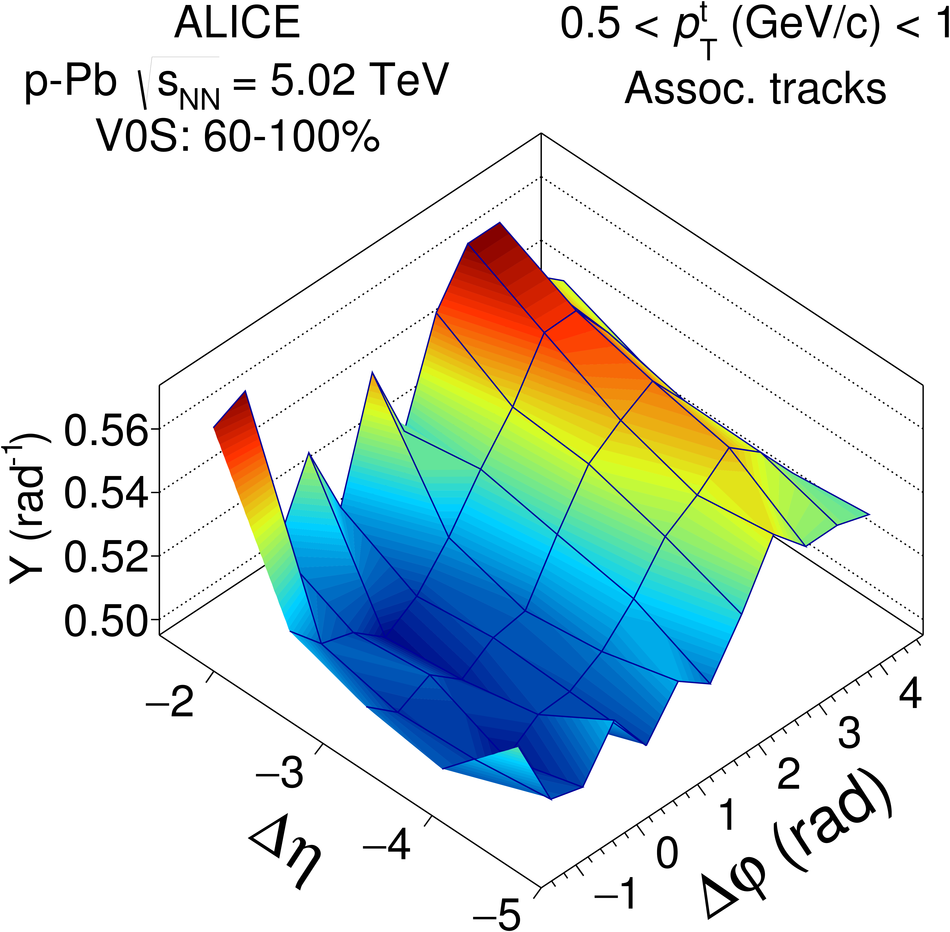# Forward-central two-particle correlations in p-Pb collisions at $\sqrt{s_{\rm NN}}$ = 5.02 TeV

Two-particle angular correlations between trigger particles in the forward pseudorapidity range ($2.5 <~ |\eta| <~ 4.0$) and associated particles in the central range ($|\eta| <~ 1.0$) are measured with the ALICE detector in p-Pb collisions at a nucleon-nucleon centre-of-mass energy of 5.02 TeV. The trigger particles are reconstructed using the muon spectrometer, and the associated particles by the central barrel tracking detectors. In high-multiplicity events, the double-ridge structure, previously discovered in two-particle angular correlations at midrapidity, is found to persist to the pseudorapidity ranges studied in this Letter. The second-order Fourier coefficients for muons in high-multiplicity events are extracted after jet-like correlations from low-multiplicity events have been subtracted. The coefficients are found to have a similar transverse momentum ($p_{\rm T}$) dependence in p-going (p-Pb) and Pb-going (Pb-p) configurations, with the Pb-going coefficients larger by about $16\pm6$%, rather independent of $p_{\rm T}$ within the uncertainties of the measurement. The data are compared with calculations using the AMPT model, which predicts a different $p_{\rm T}$ and $\eta$ dependence than observed in the data. The results are sensitive to the parent particle $v_2$ and composition of reconstructed muon tracks, where the contribution from heavy flavour decays are expected to dominate at $p_{\rm T}>2$ GeV/$c$.

Figures

## Figure 1

 Parent particle composition of reconstructed muon tracks (left panel) and reconstruction efficiency for muons from pion and kaon decays relative to that for heavy flavor (HF) decay muons (right panel) from a detector simulation of the ALICE muon spectrometer## Figure 2

 Associated yield per trigger particle as a function of $\Deta$ and $\Dphi$ for muon-track correlations in p-Pb~(left) and muon-tracklet correlations in p-Pb (middle) and Pb-p (right panels), measured in 60-100% (top row) and 0-20% (bottom row) event classes The trigger particle (muon) range is $0.5< \pt^a< 1$ GeV/$c$, the associated particle intervals are $0.5< \pt^a < 4.0$ GeV/$c$ for tracks and $0< \Delta\varphi_{\,\rm h}< 5$ mrad for tracklets Statistical uncertainties are not shown## Figure 3

 Top panels: Associated yield per trigger particle as a function of $\Dphi$ and $\Deta$ for muon-track correlations in p-Pb (left) and muon-tracklet correlations in p-Pb (centre) and Pb-p (right) collisions for the 0-20% event class, where the corresponding correlation from the 60-100% event class has been subtracted. Statistical uncertainties are not shown. The trigger particle (muon) range is $0.5< \pt^t< 1$ GeV/$c$, the associated particle intervals are $0.5< \pt^a< 4.0$ GeV/$c$ for tracks and $0< \Delta\varphi_{\,\rm h}< 5$ mrad for tracklets Bottom panels: The same as above projected onto $\Dphi$. The lines indicate the fit to the data and the first harmonic contributions as explained in the text## Figure 4

 Comparison of $v^{\mu}_2${2PC,sub} for $-4< \eta< -2.5$ extracted from muon-track and muon-tracklet correlations in p-Pb collisions at $\snn=5.02$ TeV## Figure 5

 The $v^{\mu}_2${2PC,sub} coefficients from muon-tracklet correlations in p-going and Pb-going directions (left) and their ratio (right) for $-4< \eta< -2.5$ in p-Pb collisions at $\snn=5.02$ TeV. The data are compared to model calculations from AMPT